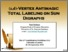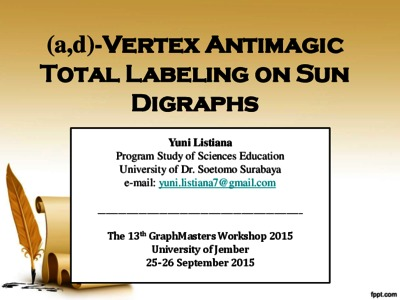# On (a; d)-Vertex Antimagic Total Labeling of Sun Digraph

Listiana, Yuni (2015) On (a; d)-Vertex Antimagic Total Labeling of Sun Digraph. In: The 13th Graph Masters Workshop, 25-16 September 2015, University of Jember, Indonesia.Preview
Text
Master Graph Yuni Presentasi.pdf - Presentation

Download (901kB) | Preview

## Abstract

Abstract. Digraph D(V; A) is a graph which each of edge has orientation called arc. Notice that a set of vertices and a set of arcs on D, respectively, is denoted by V and A. The sum of vertices on D is called as order of D and denoted by jV j, while the sum of arcs on D is called as size of D and denoted by jAj. If jV j = s and jAj = r, then the (a; d)-vertex antimagic total labeling of digraph D de�ne as a bijection function of � from the set of vertices V and the set of edges A to the integers f1; 2; 3; :::; s + rg such that the total weight of vertices form an arithmetical progression a; a + d; a + 2d; :::; a + (s 1)d, with total weight of vertex is Wt(v) = �!uv; u2A+(v)(!uv) + �(v) �z2A(v)! uv 2 A, d � 0, and a,d is integer. In this paper, we construct an (a; d)-vertex magic total labeling on sun digraph. Sun digraph,! , n � 3, is de�ned as cycle digraph by adding an orientation pendant at each vertex of the cycle. The orientation of arcs in the sun digraph follow clockwise direction. The result of this paper is a construction of (a; d)-vertex magic total labeling on M n ! M , n � 3, for d = 0; 1; 2; 3; 4. Beside that, we also have a result that is there are no super (a; d)-vertex antimagic total labeling n on! Mn, n � 3.�(!vz), for

Item Type: Conference or Workshop Item (Keynote) L Education > L Education (General)Q Science > Q Science (General) Fakultas Keguruan dan Ilmu Pendidikan Lambang Probo Sumirat 27 Oct 2016 05:33 27 Oct 2016 05:33 http://repository.unitomo.ac.id/id/eprint/53

### Actions (login required)View Item